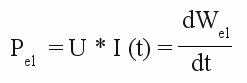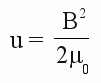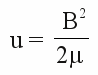# Energy stored in magnetic fields

## Electric energy

We recognized electric power at formula [3.8] being the product of voltage and current. Corresponding to the mechanical work, electrical work is the product of power and time:

[3.38]Where is:
Wel - electrical work, Pel - electrical power, U - voltage, I - current, t - time

Ohmic resistors convert electric energy into thermal energy. Equation [3.38] shows that the "roasted" amount of energy is proportional to the time (while voltage and thereby current are constant over time).
We need electric energy to create a magnetic field around an inductor. Let's have a look at an inductor without ohmic resistance: While the input voltage is constant, the current through the inductor is in no way constant. With the correlation:With the help of formula [3.29] of induced voltage we get:Short form:

[3.39]Where is:
Wel - electric work respectively energy, Pel - electric power, I - current, U - voltage, L - Inductance, t - time

Energy doesn't disappear somewhere inside the Nirvana, but is always converted into another form. A capacitor converts the input energy into an electric field, wherein charged particles feature potential energy. Ohmic resistors convert electric energy into thermal energy (=kinetic energy of atoms). What happens to the electric energy applied to the inductor?
Well, those energy is converted completely into a magnetic field carrying the energy (we neglected ohmic resistance). That's not easy to understand, because in practice it's hard to store and release energy in a magnetic field. If we connect a capacitor to a battery, a current runs into the device until it is charged. We can disconnect the capacitor from the battery and even hours later we can measure the battery voltage at the contacts of the capacitor or we can use the energy to power an electric motor or a small lamp.
If we connect an inductor to a battery, a magnetic field is created, carrying electric energy. We can't simply disconnect the inductor from the battery to keep the magnetic field and thereby the energy inside of the inductor. To keep the magnetic field the current running through the inductor has to be constant (see chapter induction and self-induction). When disconnecting the inductor from the battery we have to short-circuit the clamps of the inductor simultaneously! That is not easy to realize and even if we do so, the magnetic field won't be conserved for hours. The current running through the wire of the inductor is converted into thermal energy because of the ohmic resistance. The lossy process of discharging an inductor as described at formula [3.36] and [3.37] occurs.
To be able to keep the stored energy in an inductor for an arbitrary time span we must use a conducting material without resistance. "Superconductors" like that have to be cooled down to temperatures below -140°C.

## Energy in magnetic fields

The energy density of any point of a magnetic field in a vaccum is given by:

[3.40]For linear, non-dispersive materials (such that B = μ * H is given) we get:

[3.41]Where is:
u - magnetic energy density, B - magnetic flux density,
μ0 - vacuum permeability (=magnetic constant), μ - permeability of the material

The total energy of the magnetic field is given by the sum of the energy density of the single points. For this reason the energy of a magnetic field shifts while:
1.) the energy density is altered.
2.) the volume of the magnetic field is modified.

Figure 1:
The energy stored in a magnetic field decreases while the current through the windings of an inductor is reduced. At the drawing this fact is visualized by a lower number of magnetic field lines, indicating lower flux density.
Magnetic energy is converted into electric energy during this process. Inside of a not idealized inductor, those electric energy is converted into thermal energy.
Figure 2:
Total energy of two permanent magnets:
At the top of the illustration two bar shaped magnets point with their north poles to each other, at the middle the north pole of the left magnet points to the south pole of the right magnet. The overall density of field lines inside of the red marked area is higher at the top than at the middle. Accordingly the total energy of the magnetic fields is higher while the north poles are pointing to each other. While rotating the left magnet for 180 degrees, magnetic energy is converted into mechanical energy. Mechanical work can be done by this process.
The distance between the two magnets is reduced at the bottom of the drawing. For this reason the flux density between the two magnetic poles (red circle) increases slightly, but the effect of the decreasing volume overbalances and the magnetic energy decreases again. While reducing the gap between the magnets, mechanical work is done. The magnets attract each other.
Figure 3:
Magnetic field of an inductor with an u-shaped iron core:
When connected to a DC-voltage, a magnetic field being similar to those of a u-shaped permanent magnet is created.
An iron bar is brought to the u-shaped magnet at the middle drawing. The density of the field lines is increasing between the poles of the magnet and the iron bar, but this effect is overbalanced by the decreasing volume of the magnetic field. The energy stored in the magnetic field is reduced. While the iron bar is moving closer to the electromagnet the volume of the magnetic field is reduced again. The energy of the magnetic field decreases but the potential energy of the lifted iron bar increases..